Search

# Derivatives and differentiation tutorial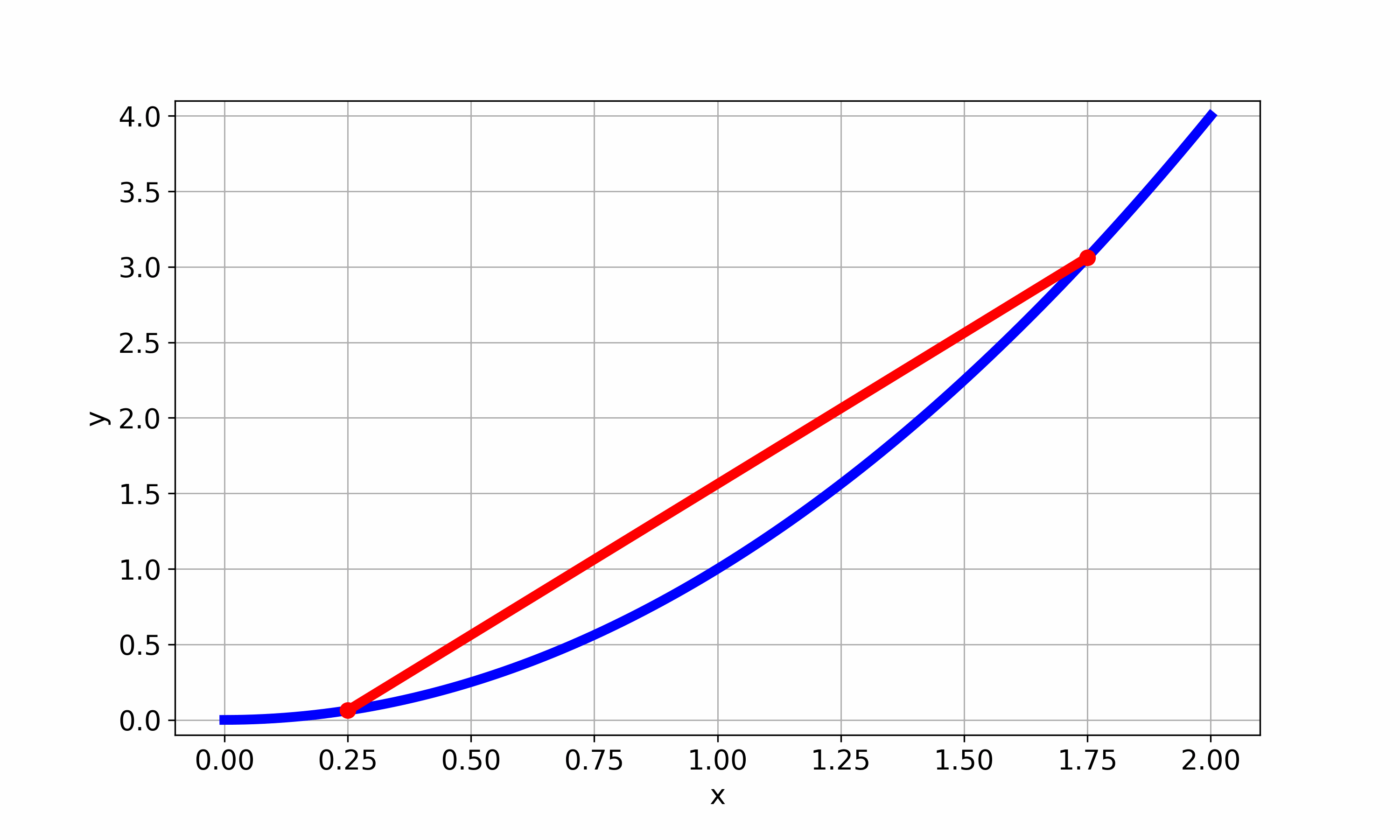Key concepts: Derivatives/differentiation Product rule Chain rule Quotient rule If you are reading this tutorial, your teacher has probably told you about different ways to take "derivatives" of functions and given you a whole list of expressions to differentiate. You are here because you have no clue which method to use or how to get started. Don't worry, we've got you covered! Question 1: Find the derivative ofusing the "product rule" Hmm, how should we go about doing this? STEP 1: MAKE A GRAPH OF THE FUNCTION You can use whatever graphing program you like (the one below was made by typing the expression in to the google search bar).Just promise me something: Always, always, always, always, ALWAYS make a graph when your teacher gives you a function and asks you to do anything to it! Why? I know it seems like extra work, and trust me, I am just as lazy as you are. The reason is that the expressiontells you literally nothing about how the function behaves. Put your hand over the graph I just made, look only at the mathematical expression and tell me the following: How many maxima does the function have? How many minina? Does it become bigger or smaller if we plug in a larger x-value? Is it always positive, or does it sometimes become negative? Making a graph can tell you all of this information instantly. You will still need to prove the answers mathematically. The graph makes this way easier because you actually know what you are looking for. Anyways, that is enough of me ranting. The point is that we can use graphs to make predictions about the final result:

• The derivative is positive but small for very negative x

• It becomes zero when the function peaks

• It then gets negative

• It becomes zero at x=0

• It gets very positive after that.

Let us check if these predictions are correct. STEP 2: LOOK AT THE FUNCTION In this case, the whole function clearly consists of two smaller functions multiplied together.This tells us that we should use the product rule. It states that if: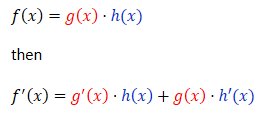For our function:Does this match the predictions we made?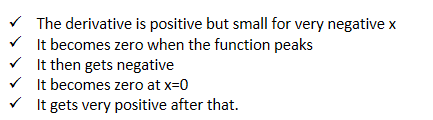We can even go crazy and take another derivative:Feel free to keep going. I am not going to stop you from getting smarter! Question 2: Find the derivative ofusing the "chain rule". STEP 1: GRAPH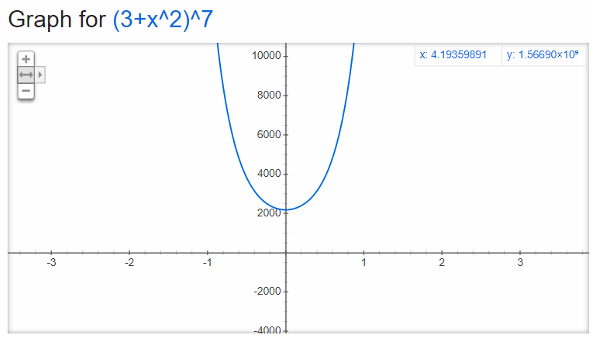We can use the graph to make the following predictions:STEP 2: LOOK AT THE FUNCTION

What do we notice about the function?Well, it seems to consist of one small function packaged inside of a bigger one:The fact that we have one function inside of another one tells us that we can use the chain rule:For this function:Let us check if the predictions are correct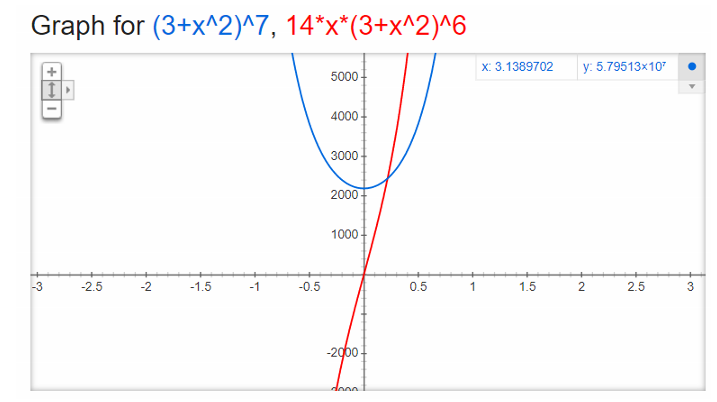Let's go even crazier and take another derivative:What do we do now? We have two functions multiplied together, but one of them is one of those nasty "function inside another function"-functions.